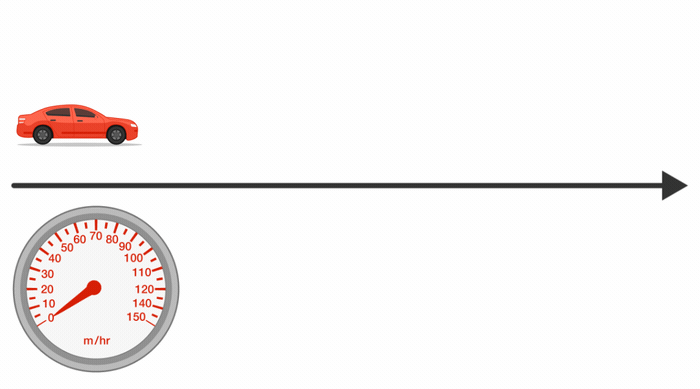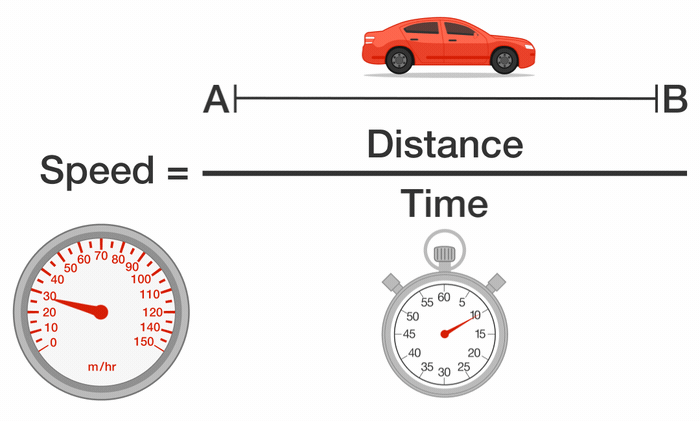### Calculus Fundamentals

The physical world is changing all the time: cars accelerate, planets move around the sun, economies fluctuate. In order to study these changing quantities, a new set of tools was developed in the 17th century. Calculus is the mathematical study of things that change.Calculus can be tricky, but the key ideas behind it are intuitive and beautiful. In this course, we’re going to look at those ideas, and get a firm conceptual and practical understanding of this new way of looking at the changing physical world.

# Indeterminate Forms

To appreciate the subtleties involved in things that change, first imagine you’re in a car driving at a steady 30 miles an hour. Since speed is $\frac{\textrm{distance}}{\textrm{time}},$ it’s pretty clear exactly what that means: every hour you cover 30 miles. In a half-hour you cover 15 miles. In one minute, you cover $\frac{1}{2}$ a mile.

Now suppose you’re accelerating as in the image below, so the speedometer is steadily increasing. You look at the speedometer at one particular moment while still accelerating, and see that it says 30 mph. What does that physically mean?# Indeterminate Forms

We’ve stumbled on an important idea: When your speed is changing, it’s not at all clear what your speed at a single moment means! We can’t just define it as “distance divided by time”. What time interval could we use? 1 second? 0.001 second? In the previous situation, no matter how small the interval, for most of it the car will be traveling greater than 30 mph, so $\frac{\textrm{distance}}{\textrm{time}}$ will be greater than 30.

Which of these might be a reasonable way to define the speed at a moment?

A) $\frac{\textrm{distance}}{\textrm{time}}$ where the time interval is the smallest positive number possible, i.e. “the next biggest number” after 0.

B) $\frac{\textrm{distance}}{\textrm{time}}$ where the time interval is actually 0.

C) We calculate $\frac{\textrm{distance}}{\textrm{time}}$ for positive time intervals, but we do this repeatedly as the time intervals get smaller and smaller, and see whether $\frac{\textrm{distance}}{\textrm{time}}$ is approaching something.

# Indeterminate Forms

When your speed is changing, the idea of “speed at a single moment” needs to be defined by a limiting process. We look at $\frac{\textrm{distance}}{\textrm{time}}$ for small time intervals containing the instant in question, and allow those intervals to get smaller and smaller. (For example, we could look at 1 second, 0.1 seconds, 0.01 seconds, etc.) If, as the time interval goes down to 0, $\frac{\textrm{distance}}{\textrm{time}}$ approaches some number $s,$ we define the speed at the single moment to be $s.$

Now, as the time interval gets smaller, so does the distance travelled. So as the denominator gets closer to 0, so does the numerator. We will refer to this situation as a “$\frac{0}{0}$ indeterminate form”. We’re not actually dividing 0 by 0 here--that’s illegal. Instead, we’re looking at an expression as its numerator and denominator both approach 0. Roughly speaking, if the numerator approaches 0 thirty times faster than the denominator does, the result of this limiting process will be 30 mph. (That is, as the time approaches $0,$ if $\text{distance} = 30 \times \text{time} ,$ then $\text{distance} / \text{time} = 30.)$ It’s like a race between the top and the bottom!# Indeterminate Forms

Let’s turn to a different question. Suppose again that our speed is changing; for example, maybe we’re accelerating at a constant rate from $30$ mph to $40$ mph in $1$ minute.

How do we calculate the distance traveled between $t=0$ and $t=1?$

We'll need a function describing how velocity (in miles per minute) is changing over time $t$ (in minutes). Given that $30$ miles per hour is $\frac{1}{2}$ mile per minute and $40$ miles per hour is $\frac{2}{3}$ miles per minute, and given a constant acceleration, we can determine the linear equation passing through the points $\left(0,\frac{1}{2}\right)$ and $\left(1,\frac{2}{3}\right).$ This equation is $v(t) = \frac{t}{6} + \frac{1}{2}.$

If the speed were constant, we’d just say $\textrm{distance} = \textrm{speed} \times \textrm{time}.$ But since the speed is changing, we need a new idea, which we’ll look at on the next page. The best we can do now is to place bounds on the answer.

What can we say about the distance traveled when accelerating from 30 mph to 40 mph in 1 minute? Pick the tightest interval possible. (Hint: what would be the distance if we were traveling at 30 mph the whole minute?)

# Indeterminate Forms

If the speed were constant, we could just say $\textrm{distance} = \textrm{speed} \times \textrm{time}.$ Here’s the new idea. Take the time interval (0 to 1), and chop it up into 5 pieces: $0 \leq t \leq \frac{1}{5},$ $\frac{1}{5} \leq t \leq \frac{2}{5},$ etc. On each piece, pretend the speed doesn’t change. So pretend the speed is $s_1$ (which is 30 mph) on the whole first piece, and $s_2$ (which is a little more than 30 mph) on the whole second piece, etc.

On each piece, we calculate the distance traveled during that piece, which is speed $\times$ time (where time for each piece is $\frac{1}{5}$), and then we add all 5 distances together. So we have $\frac{1}{5}s_1 + \frac{1}{5}s_2 + \ldots .$

Of course, the speed isn’t actually constant over each piece. This is an approximation - but it's better than our last one. To get an even better approximation we can repeat the process by chopping the time interval up into smaller pieces, say 100 pieces. And then 1000, and then 10,000. We look at what happens to the sum of all the little distances as the number of pieces $n$ gets larger.

Let's consider (for the purposes of this problem) "value that approaches something infinitely large" to be $\infty$ and "value that approaches something infinitely small" to be $0 .$ Considering the expression for distance and assuming speed is always positive, what arithmetic expression do you think the limiting process corresponds to? In other words, as $n$ gets very large, what indeterminate form best represents $\text{distance} = \frac{1}{n} \times \sum\limits_{j=1}^{n} s_{j}?$

# Indeterminate Forms

When things change, we usually need limiting processes to describe them. When we try to define speed at a particular moment, we find the $\frac{0}{0}$ indeterminate form: a limiting process of fractions where both numerator and denominator approach 0. When we try to calculate distance given a changing speed, we find the $\infty \times 0$ indeterminate form: a limiting process of adding up more and more numbers, as the numbers themselves are approaching 0.

And this was just looking at the speed of a car along a straight line. You can imagine that these ideas are even more important with more complex forms of change in the natural world. A physicist needs them to understand the movement of the planets about the sun; an engineer needs them to understand the flow of current in a circuit; a biologist needs them to model changing animal populations.

“Calculus” comes from the same root as “calculate”, and calculus is just a tool that allows us to calculate these indeterminate forms with ease and confidence. The $\frac{0}{0}$ form is the basis of differentiation, which we’ll study later in the course; the $\infty \times 0$ form is the basis of integration.

First, we have to get a better grasp on the idea of a “limiting process” that lies behind both forms. That’s where we’ll start.

×